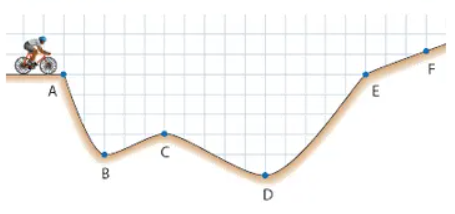# Problem: A bicyclist is stopped at the entrance to a valley, as sketched below: A. Where would the bicyclist have the highest potential energy? B. Where would the bicyclist have the lowest potential energy? C. Where would the bicyclist have the highest kinetic energy? D. Where would the bicyclist have the highest speed? E. Would the bicyclist's kinetic energy be higher at B or C? F. Would the bicyclist's potential energy be higher at B or C? G. Would the bicyclist's total energy be higher at B or C? H. Suppose the bicyclist lets off the brakes and coasts down into the valley without pedaling. Even if there is no friction or air resistance to slow him down, what is the farthest point the bicyclist could reach without pedaling?

###### FREE Expert Solution

Recall:

$\overline{){\mathbf{P}}{\mathbf{.}}{\mathbf{E}}{\mathbf{.}}{\mathbf{=}}{\mathbf{mgh}}}$

$\overline{){\mathbf{K}}{\mathbf{.}}{\mathbf{E}}{\mathbf{.}}{\mathbf{=}}\frac{\mathbf{1}}{\mathbf{2}}{{\mathbf{mv}}}^{{\mathbf{2}}}}$

100% (118 ratings)###### Problem Details

A bicyclist is stopped at the entrance to a valley, as sketched below:A. Where would the bicyclist have the highest potential energy?

B. Where would the bicyclist have the lowest potential energy?

C. Where would the bicyclist have the highest kinetic energy?

D. Where would the bicyclist have the highest speed?

E. Would the bicyclist's kinetic energy be higher at B or C?

F. Would the bicyclist's potential energy be higher at B or C?

G. Would the bicyclist's total energy be higher at B or C?

H. Suppose the bicyclist lets off the brakes and coasts down into the valley without pedaling. Even if there is no friction or air resistance to slow him down, what is the farthest point the bicyclist could reach without pedaling?